# Euler totient function

This article defines an arithmetic function or number-theoretic function: a function from the natural numbers to a ring (usually, the ring of integers, rational numbers, real numbers, or complex numbers).
View a complete list of arithmetic functions

## Definition

Letbe a natural number. The Euler phi-function or Euler totient function of, denoted, is defined as following:

• It is the order of the multiplicative group modulo, i.e., the multiplicative group of the ring of integers modulo.
• It is the number of elements inthat are relatively prime to.

### In terms of prime factorization

Suppose we have the following prime factorization of:.

Then, we have: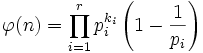.

In other words: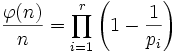.

## Properties

Property Satisfied? Statement with symbols
multiplicative function Yes Ifandare relatively prime natural numbers, then.
completely multiplicative function No It is not true for arbitrary natural numbersandthat. For instance, if, then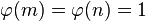whereas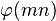is 2.
divisibility-preserving function Yes Ifandare natural numbers such thatdivides, then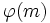divides.

## Behavior

### High and low points (relatively speaking)

• Primes are high points: We also have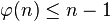for. Equality occurs if and only ifis a prime number.
• Primorials are low points: Roughly, the numbers occurring as primorials (products of the first few primes) have the lowest value ofrelative to, compared with other similarly sized numbers.

### Measures of difference

We use the infinitude of primes for arguing about limit superiors. The limits discussed are in the limit as. Note that the last quotient is undefined for.

Measure Limit superior Explanation Limit inferior Explanation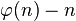-1 maximum value of -1 occurs at primes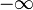Consider the sequence of powers 2., so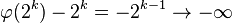.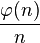1 At each prime, value is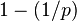. Limit is 1 as0 Consider the sequence of primorials. The corresponding values of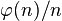are products of the valuesfor the first few primes. The limit of these is the infinite product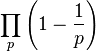over all prime. This diverges because the infinite sum of the reciprocals of the primes diverges.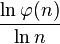1 For each prime, the limit is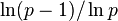,which approaches 1. 1 We can show that for every, there exists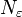such that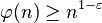for all.

## Summatory function and average value

### Summatory function

The summatory function of the Euler phi-function is termed the totient summatory function.

## Relation with other arithmetic functions

### Similar functions

• Universal exponent (also called Carmichael function) is the exponent of the multiplicative group modulo. The universal exponent of, usually denoted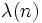, divides.
• Dedekind psi-function is similar tothe Euler phi-function, and is defined as: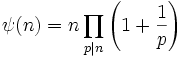.

### Relations expressed in terms of Dirichlet products..

•: In other words, the Dirichlet product of the Euler phi-function and the divisor count function equals the divisor sum function: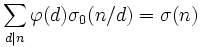.

•.

### Inequalities

•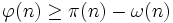: Here,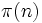is the prime-counting function, and counts the number of primes less than or equal to, while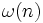is the prime divisor count function of.
•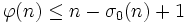: Here,is the divisor count function, counting the total number of divisors of.

## Relation with properties of numbers

• Prime number: A natural numbersuch that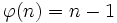.
• Polygonal number: A natural numbersuch thatis a power of, or equivalently, such that the regular-gon is constructible using straightedge and compass.

## Dirichlet series

Further information: Formula for Dirichlet series of Euler phi-function

The Dirichlet series for the Euler phi-function is given by:.

Using the Dirichlet product identity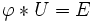and the fact that Dirichlet series of Dirichlet product equals product of Dirichlet series, we get: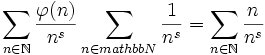.

This simplifies to: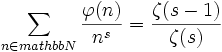.

This identity holds not just for the formal Dirichlet series, but also for their analytic continuations, and is valid universally for the meromorphic functions.

## Algebraic significance

The Euler phi-function ofis important in the following ways:

• It is the number of generators of the cyclic group of order.
• It is the order of the multiplicative group of the ring of integers modulo(in fact, this multiplicative group is precisely the set of generators of the additive group).